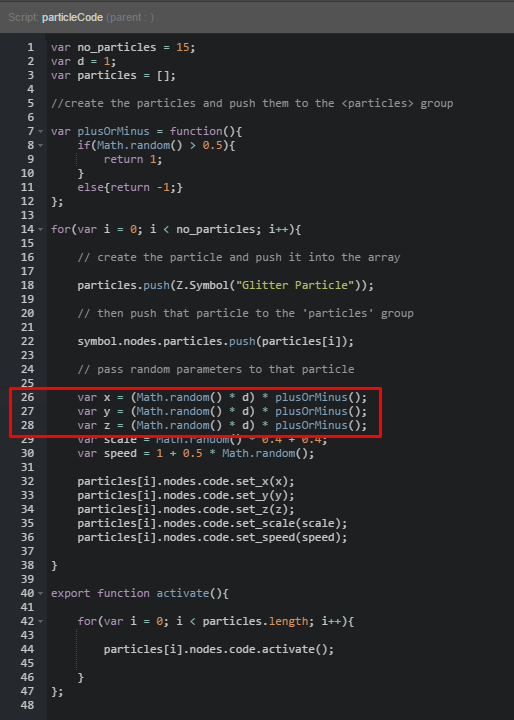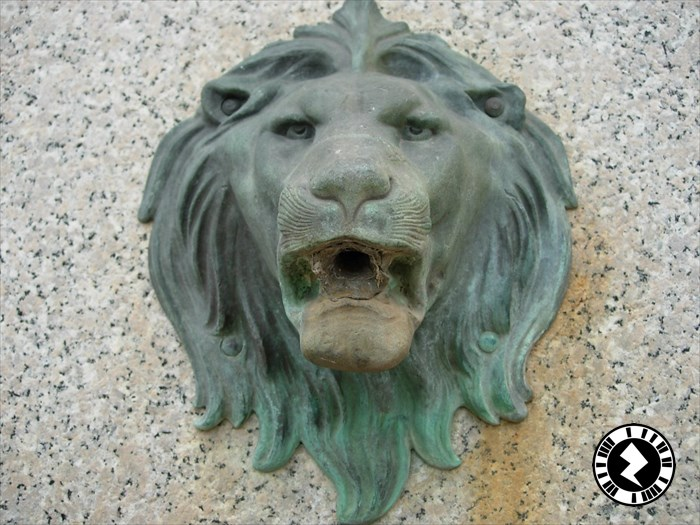Help in particle

I would like to know if you have some examples of how to create particle effects in ZapparStudio?

1 Like

Hi There,

There is no built in particle effects at this point in time in Studio, however, our in-house creative team has built one for use across their projects which we’re happy to share with youThe project below has a plane in it acting as a button that when pressed activates some code that randomly positions and moves a collection of star burst images. You can enter the ‘Glitter Particle’ subsymbol if you want to dig deeper into the implementation.

Particle Effect.zpp (283.4 KB)

Hope this is usefulMark

6 Likes

Hay Mark,

I’m playing with your Particle Effect and I’m loving it!!
I have a question. When I run your code it doesn’t change the pattern each time I press the button.
How would I get it to do that?

Thanks,
Steve

1 Like

Hi Steve,

The plane just calls the activate function, it only randomizes one time at the begging and then uses those values each subsequent time. You can move the for loop inside the activate function in the particleCode script to randomize everytime. So it would look like the following:

var no_particles = 15;
var d = 1;
var particles = [];

//create the particles and push them to the <particles> group

var plusOrMinus = function(){
if(Math.random() > 0.5){
return 1;
}
else{return -1;}
};

export function activate(){

for(var i = 0; i < no_particles; i++){

// create the particle and push it into the array

particles.push(Z.Symbol("Glitter Particle"));

// then push that particle to the 'particles' group

symbol.nodes.particles.push(particles[i]);

// pass random parameters to that particle

var x = (Math.random() * d) * plusOrMinus();
var y = (Math.random() * d) * plusOrMinus();
var z = (Math.random() * d) * plusOrMinus();
var scale = Math.random() * 0.4 + 0.4;
var speed = 1 + 0.5 * Math.random();

particles[i].nodes.code.set_x(x);
particles[i].nodes.code.set_y(y);
particles[i].nodes.code.set_z(z);
particles[i].nodes.code.set_scale(scale);
particles[i].nodes.code.set_speed(speed);

}

for(var i = 0; i < particles.length; i++){

particles[i].nodes.code.activate();

}
};

Edit* I’ve just realized that this is creating a new set of particles each time which is bad as it will build up and slow your phone down. What you will want to do is create your pool of particles outside of the activate function and then change their x,y,z, scale and size inside activate.

Thanks,
Mark

1 Like

Thanks Mark,

I was thinking I would have a problem with build up of new sets. I didn’t see how to remove a new item. Can we? or would I have to remap x,y,z? I’m looking at using this on other things later.

Thanks,
Steve

1 Like

We don’t have any garbage collection in Studio at the moment so it’s best to just use a pool of objects. It’s done easily enough by moving the random number generation to the activate function but keeping the creation of the particles to the for loop that runs once:

for(var i = 0; i < no_particles; i++){

// create the particle and push it into the array

particles.push(Z.Symbol("Glitter Particle"));

// then push that particle to the 'particles' group

symbol.nodes.particles.push(particles[i]);

}

export function activate(){

for(var i = 0; i < particles.length; i++){

// pass random parameters to that particle

var x = (Math.random() * d) * plusOrMinus();
var y = (Math.random() * d) * plusOrMinus();
var z = (Math.random() * d) * plusOrMinus();
var scale = Math.random() * 0.4 + 0.4;
var speed = 1 + 0.5 * Math.random();

particles[i].nodes.code.set_x(x);
particles[i].nodes.code.set_y(y);
particles[i].nodes.code.set_z(z);
particles[i].nodes.code.set_scale(scale);
particles[i].nodes.code.set_speed(speed);

particles[i].nodes.code.activate();

}

};

Mark

1 Like

Thank you Mark.

I’m looking at doing something with moving rocks but I did’t see how to clean up things.
What would be the best way to track how many pooled objects I have. (for debugging)?

Steve

1 Like

Hi Steve,

Once constructed there’s no way at the moment for “deleting” the memory used so it’s not possible to do the traditional clean up of removing objects.

The best thing to do is create an array of the objects like we do in the example above with the particles[] array. You can use the .length property of the array to tell how many objects you have.

Thanks,
Mark

1 Like

Thank you!

I was playing with the Particles code and found it had colored one. So I added your code and a random color code to it:

Steve

var no_particles = 15;
var d = 1;
var particles = [];

//create the particles and push them to the group

var plusOrMinus = function(){
if(Math.random() > 0.5){
return 1;
}
else{return -1;}
};

// Color pick send color number 0-4 if more then 3 change

var color = function(){
var c = 0;
c = Math.floor(Math.random() * 11);

while (c > 5)
{
c = Math.floor(Math.random() * 11);

};
return c;

};

for(var i = 0; i < no_particles; i++){

// create the particle and push it into the array

particles.push(Z.Symbol(“Glitter Particle”));

// then push that particle to the ‘particles’ group

symbol.nodes.particles.push(particles[i]);

}

export function activate(){

for(var i = 0; i < no_particles; i++){

// pass random parameters to that particle

var x = (Math.random() * d) * plusOrMinus();
var y = (Math.random() * d) * plusOrMinus();
var z = (Math.random() * d) * plusOrMinus();
var scale = Math.random() * 0.4 + 0.4;
var speed = 1 + 0.5 * Math.random();

particles[i].nodes.code.set_x(x);
particles[i].nodes.code.set_y(y);
particles[i].nodes.code.set_z(z);
particles[i].nodes.code.set_scale(scale);
particles[i].nodes.code.set_speed(speed);
particles[i].nodes.code.set_color(color());

}

for(var i = 0; i < particles.length; i++){

particles[i].nodes.code.activate();

}
};

1 Like

This is -almost- exactly what I’m trying to do, and I’ve been diving into it to find out how to change it to suit my needs.
What I’d like to do is instead of having the particles radially move outward from their random position a random distance away from 0,0, but in the same plane, I’d like them to move -up- (perpendicular from the plane). I can’t seem to find this in the code, the speed is how fast it goes, not in which direction, so I figure this is ‘hardcoded’ within the Glitter Particle.

I can’t find how to change it though. Can you give me some pointers?

1 Like

Hi Mark,

In the ‘particleCode’ script node within the zpp you should see three variables: x, y and z.These variables are currently being assigned a randomised value through the Math.random() function, and then being passed in to the set_x, set_y, and set_z functions (exported from the ‘Glitter Particle’ subsymbol) later on in the script.

By changing these values, you can change the direction that the particles will be travelling towards.

Hope this helps.

All the best,
Seb

1 Like

Thanks, Seb!

I assumed those were the starting points of the particle. I Should be able to figure it out now1 Like

I have tried my own particles system, “water” style…5 Likes

Very cool!

1 Like

Here it’s the zpp file for those who want to play with it. Code similar from Mark’s, but the nodes are managed with JS objects. Everything is inside the node “code”. Enjoy!Particles.zpp (370.4 KB)

3 Likes

Many thanks!
Cheers!

1 Like

This is great, thanks for sharing! Are you looking to use this is a project soon? Love to hear a little more about what your plans are1 Like

Glad you like it!Yes I’m using this kind of code for a small game I’m working on - nothing serious, just a way to learn more about Studio. In fact, I’m starting to consider Studio as a kind of IDE pour AR/VR JS application!2 Likes

Hi Mark,

I have limited experience with coding- could you modify this code so that instead of activating when the box is pressed, the code will attach to an imported fbx object ( that has an attached animation) and will activate when the fbx object is pressed?

1 Like

What your asking for is more or less the same thing. You have to use a button on any models. the model it’s self does not have a clicked on event. Place the button inside your model and change it to not visible.

Steve